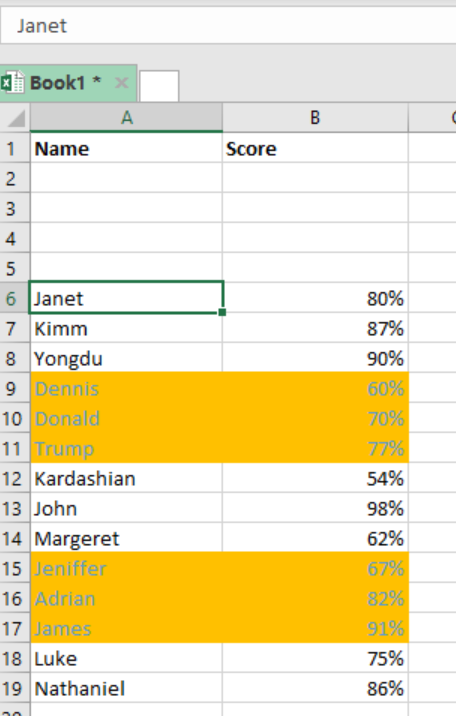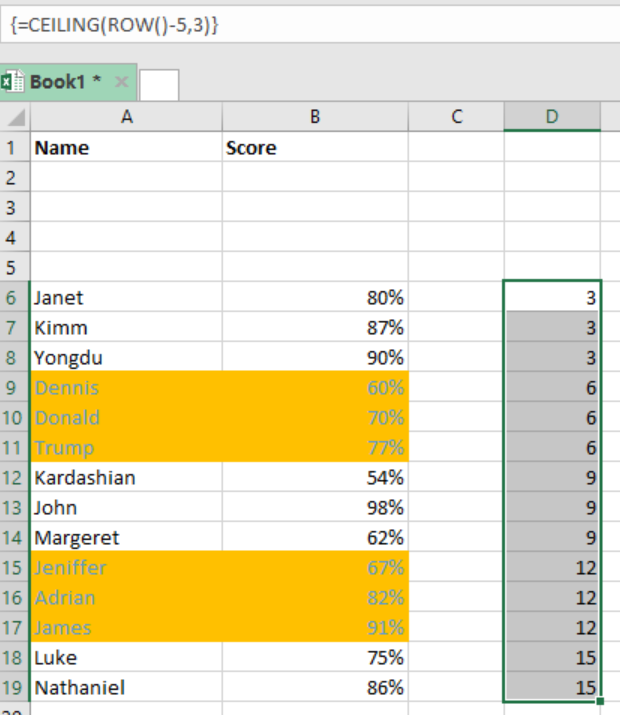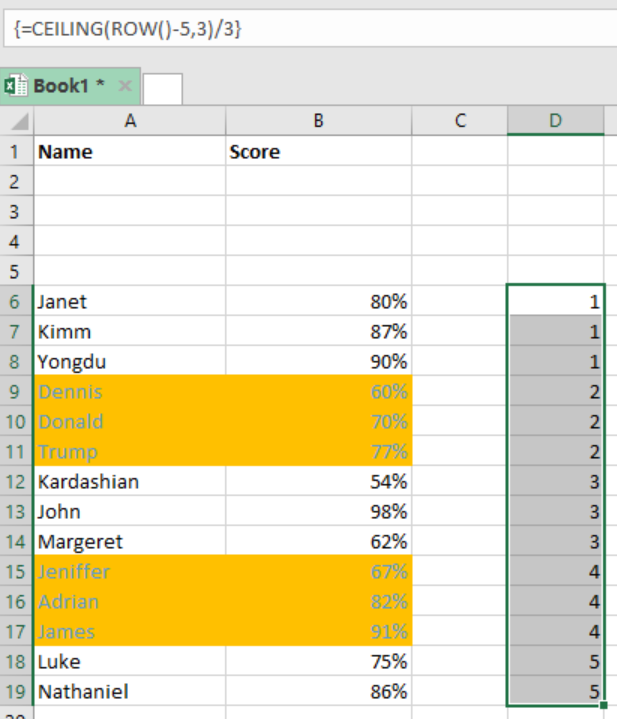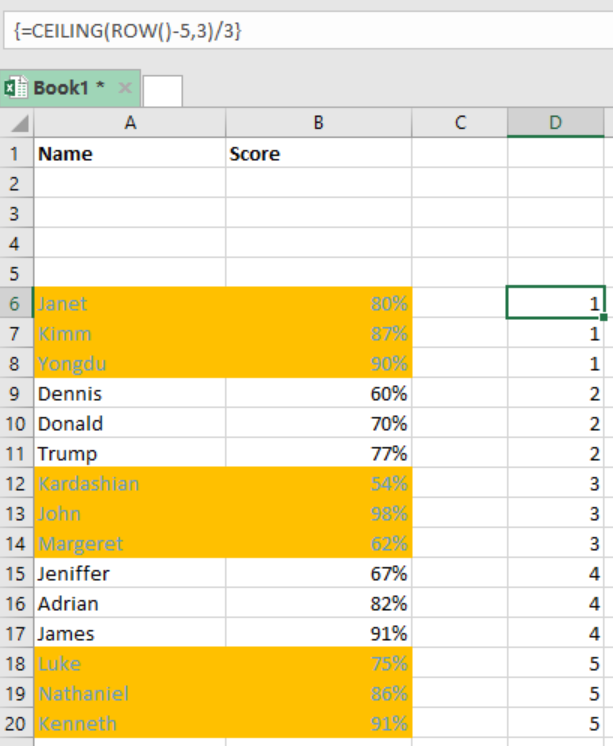Get instant live expert help with Excel or Google Sheets“My Excelchat expert helped me in less than 20 minutes, saving me what would have been 5 hours of work!”

#### Post your problem and you’ll get Expert help in seconds.

Your message must be at least 40 characters
Our professional Expert are available now. Your privacy is guaranteed.

# Shade alternating groups of n rows

When we want to shade every n rows in excel, we can use a formula that is based on ROW, CEILING and ISEVEN functions together with conditional formatting. This article provides a clear guide on how you can shade alternating groups of n rows while working in excel.Figure 1: Shading every n rows in excel

## General syntax of the formula

`=ISEVEN(CEILING(ROW() –offset, n)/n)`

Where;

• n- is the number of rows in a group
• offset- is the number used to normalize the first row

## How this formula works

This formula is composed of three functions, and they play the following functions:

• ROW function- this is used to normalize the row numbers to start with 1
ROW() – offset
Notice that the first row of our data in the figure above is in row 6, so we can use an offset of 5.
• The result of the ROW function goes to the CEILING function. This function is responsible for rounding incoming numbers up to a specified multiple of n.Figure 2: Example of how to shade alternating rows in excel

• Now to count by groups of n, which in our case is 3, the count above is divided by n, 3, starting with 1 as illustrated below;Figure 3: Illustration of how to shade alternating groups in excel

The conditional formatting is the triggered by the ISEVEN function which forces a TRUE for all the even rows in the groups. For those that are not even, the conditional formatting is not triggered, therefore, they do not change.

## How to shade first group

Instead of starting with the second, you can as well shade the first group of n rows. All you need to do is to replace the ISEVEN function with its ISODD counterpart. The formula with the example below;

`=ISODD(CEILING(ROW() –offset,n/n)`

## ExampleFigure 4: How to shade odd rows in excel

In this particular example, we have shaded all odd rows. To do this, proceed as follows;

• Step 1: Provide the data in the table
• Step 2: Highlight the entire data and apply conditional formatting.
• Step 3: While formatting, apply the formula `=ISODD(CEILING(ROW()-5,3)/3)`
• Step 4: Press “Apply and the “Ok” to apply the conditional formatting.

## Instant Connection to an Expert through our Excelchat Service

Most of the time, the problem you will need to solve will be more complex than a simple application of a formula or function. If you want to save hours of research and frustration, try our live Excelchat service! Our Excel Experts are available 24/7 to answer any Excel question you may have. We guarantee a connection within 30 seconds and a customized solution within 20 minutes.

### Did this post not answer your question? Get a solution from connecting with the expert.Another blog reader asked this question today on Excelchat:
Solution examplesI want to be able to alter the appearance (font color and fill) of a column of numbers using conditional formatting, based on being greater than or less than the numbers from another column. I am using Excel 2016. I am unable to get any formula to work. Please help!
Solved by I. J. in 59 minsI need assistance with an excel formula. I have a 12 month sheet that has a summary page on the 13 page. the monthly totals changes so on the summary page I want to sum the difference - eg. if Jan total is 1500, feb total is 1000, mar total is 800, I want the summary page to show the current amount of 800 when you place the formula to capture all months---showing the diminishing value rather than the sum of all the pages
Solved by E. L. in 60 minsI need a formula for a resource planning model that will round up if a given number is odd to the next whole number of team size.
Solved by E. E. in 22 minsin range b2:o2, I am trying to write a formula to count if the values are equal to 1 thru 27.
Solved by E. L. in 52 minsI need a range of numbers to become the number in the next column
Solved by D. F. in 47 mins## Subscribe to Excelchat.coAnother blog reader asked this question today on Excelchat: